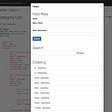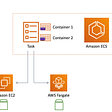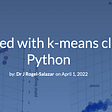# Tutorial: Data Analyzing on Chipotle Dataset with Python

This tutorial will use core Python 3.6 functions to perform some basic functions on a dataset from a day’s sale of Chipotle restaurant. I’m not going to use any additional libraries. If you know basics of python and want to see how you can use it to read data and perform some functionalities on it to get some information.

Have a look at this chipotle.tsv file of one day sales of Chipotle. This is the data on which we will perform operations like:

• Read the file with csv.reader() and store it in an object.
• Separate file into the ‘header’ and the ‘data’.
• Calculate the average price of an order.
• Create a list (or set) of all unique sodas and soft drinks that they sell.
• Calculate the average number of toppings per burrito.
• Create a dictionary in which the keys represent chip orders and the values represent the total number of orders.
• Calculate average number of items with an order.

This list can go on for countless information you can extract from a dataset. By the look of this you can see that with this one data set if you have all this information for a month’s sale you can take decisions and can also predict which stuff is likely to sell more. So let’s dive into that now.

First step which you need is to read the file using .csv reader. So you will import a library called csv

`import csv`

Because it’s a .tsv file which is similar to .csv but the only difference is that in a .tsv file the data is separated by a tab space. So the decimeter in this case will be a tab i.e. \t

Make sure the path is set to the file correctly. It depends on the editor you are using. For this example I’m using Spyder and I’ve my chipotle.tsv file is stored in the same folder as my .py file.

`with open(‘chipotle.tsv’, ‘rU’) as f:file_nested_list = [row for row in csv.reader(f, delimiter=’\t’)]`

So now we have the data in file_nested_list object. Now we can perform functions on this object.

In chipotle.tsv we have a header for the data which tells us that which column is what. So in this case we have order_id, quantity, item_name, choice_description and item_price which is the data we don’t actually need. So we will separate our header and data so that we can perform operations on data.

Using the power of indexing we will separate header and data as:

`header = file_nested_listdata = file_nested_list[1:]`

Data is everything that starts from 1 till the end of the list. Now you have the data separated from header so let’s start with the operations.

## Calculate Average price of an order

First we need to get all prices in a separate list. The because price is a string meaning \$x.xx and we need to convert it to float which means getting rid of \$ sign and assigning the value to float.

`all_prices = []for price in data:all_prices.append(float(price.replace(‘\$’,’’)))`

Because we know that price is the 5th column in the .tsv file so using zero based index we have used price and replaces \$ with empty or nothing.

As average formula is sum of all divided by total numbers of itms in the list or length of that list.

`average_price = sum(all_prices)/len(all_prices)print(‘Average price of an order: \$’,round(average_price, 2))`

We have rounded the value of average up-to two decimal points.

## Create a list (or set) of all unique sodas and soft drinks that they sell

Set always have unique items i.e. [1,2,2] list will actually mean [1,2] in the language of set. So when a customer ask for a soda it can be coke, sprite or number of other which they can have. Our data set tells us that the item number is unique meaning it is either under Canned Soda or Canned Soft Drink. So this is the point around which we will build our logic.

`unique_sodas = []for item in data:if item == ‘Canned Soda’ or item == ‘Canned Soft Drink’:unique_sodas.append(item)`

The if condition is placed on index 2 because it has the unique item_name and when it finds that we are appending out list with index 3. If you print unique_sodas it’ll be a long list but we only need to see how many sodas we have so we can convert this list to set which will automatically remove the reoccurring values from the list.

`print(set(unique_sodas))`

## Calculate the average number of toppings per burrito

This can be achieved in a number of ways but the one which we are doing is basically the simplest that can come to mind when working with basic python.

Because the number of topping has nested lists and it would be tricky to get into that so one thing which we can see is that all the values are separated by a comma. So we can count number of commas and add 1 to it and we will have the actually number of toppings.

`avg_num_topping = []for item in data:if item == ‘Burrito’:avg_num_topping.append(item.count(‘,’) + 1)`

And then the same average formula and rounding off the value:

`avg_num_per_burrito = sum(avg_num_topping)/len(avg_num_topping)print(‘Average number of toppings per burrito: ‘, int(round(avg_num_per_burrito)))`

## Create a dictionary in which the keys represent chip orders and the values represent the total number of orders

Create an empty dictionary:

`chips_dict = {}`

Index 1 which is quantity we need to add the quantity next to the name of every chips order it found.

`for item in data:if “Chips” in item:if item in chips_dict.keys():chips_dict[item] = int(chips_dict[item])+int(item)else:chips_dict[item] = item`

If Chips is in name of any index 2 field than add index 1 int value to the value of the key of that chip. If it is a chips is not added in the list create it and assign it the initial value of index 1.

`print(chips_dict)`

## Calculate the average number of items each order

Some people order drink some don’t. Every order is different so this query will answer average number of items people order. If you see the list you can see that order_id is unique and repeats only if an item is associated with the same order. So this is where our focus should be.

`avg_items_per_order = {}for item in data:if item in avg_items_per_order.keys():avg_items_per_order[item] = int(avg_items_per_order[item]+1)else:avg_items_per_order[item] = 1`

Keys in this case are order_id so this is why the if condition is on that.

`avg_value = sum(avg_items_per_order.values())/len(avg_items_per_order)print(‘Average number of items with an order: ‘,round(avg_value))`

So these are some information you can extract from a dataset. You can use the same data and think of a question about this data that interests you, and then answer it!

## More from DataCTW

DataCTW is a website of online tutorials hosted at different places and are completely free. Our aim is to provide tutorials from scratch so that anyone who is looking to learn something new can benefit from it.

## DjangoFilterBackend, SearchFilter, OrderingFilter example## Why I built Pathfix, the OAuth middleware for devs.## Imposter Syndrome as Software Engineer & How to Deal with It## How to Decide Which Features to Implement in Your App?## AMAZON ECS BEST PRACTISES## Offensive Security InfosecPrep Writeup## Create your own dataset in pandas: Music shops chain example## Getting started with k-means clustering in Python## Introduction to Python(Part-2): Python Installation